# Hotel

The rooms in the mountain hotel are double and triple. Double rooms are 25 and triple are 17 more. How many rooms are there in this hotel?

Result

s =  67

#### Solution:Leave us a comment of example and its solution (i.e. if it is still somewhat unclear...):

Showing 0 comments:Be the first to comment!#### To solve this verbal math problem are needed these knowledge from mathematics:

Do you have a linear equation or system of equations and looking for its solution? Or do you have quadratic equation?

## Next similar examples:

1. Unknown number xyzFind the number that its triple is 24. Solve by equation.
2. Tulips and daffodils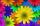Farm cultivated tulips and 211 units on 50 units more daffodils. How many spring flowers grown together?
3. School marks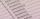Boris has a total of 22 marks. Ones have 3 times less than threes. There are two twos. How many has ones and threes?
4. Baking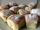There are 28 bunches, and son ate 1/2, dad ate four bunches. How many of them remain on the baking dishes?
5. Computer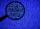A line of print on a computer contains 64 characters (letters, spacers or other chars). Find how many characters there are in 7 lines.
6. Postal stamps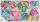Jano and Peter exchanged postal stamps. Jano gave Peter 32 stamps of the missile for 8 stamps with turtles. How good was Jano after this exchange (how many he has surplus in exchanged stamps)?
7. Bus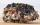On the 4-th stop take on 56 and take off 38 passengers. How many were added (write as positive number) or shrunk (write as negative number) the count of passengers?
8. CollectionMajka gave from her collection of calendars Hanke 15 calendars, Julke 6 calendars and Petke 10 calendars. Still remains 77 calendars. How many calendars had Majka in her collection at the beginning?
9. Jan and DanJan and Dan had the same money. Jan bought 5 workbooks and left him 15 CZK. Dan 6 and left him nothing. How much money have in total?
10. Number unknownAdela thought the two-digit number, she added it to its ten times and got 407. What number does she think?
11. Six te 2If 3t-7=5t, then 6t=
12. If-then equationIf 5x - 17 = -x + 7, then x =
13. Fridays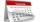Friday 13th is in 4 days. What day is today and what day it is?
14. Dividing by five and tenNumber 5040 divide by the number 5 and by number 10: a = 5040: 5 b = 5040: 10
15. Eq1Solve equation: 4(a-3)=3(2a-5)
16. FedorFedor stood in the small pool. Above the surface was a part of his body that was twice as long as part of his body underwater. What height does Fedor have, if his body part below the surface is 5 dm long?
17. I thinkI think a number. When I multiply it by five, and after that I subtract 477, I get the same number as if I multiplied it twice. What number do I think?